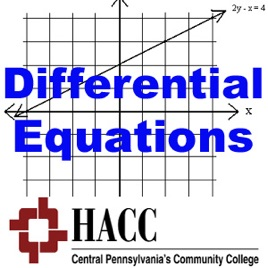238 Folgen

This course will cover ordinary differential equations of the first and second order with physical and geometrical applications; operators; the Laplace Transform; matrices; solutions in series; numerical methods.

Podcasts, in both video and pdf format, are pre-recorded with examples covering major topics presented in a Differential Equations course. Examples are chosen to help students' understanding of the concepts and the working of the methods. Software or use of graphing calculator will be included, if applicable.

Please send any suggestions/feedback to opchow@hacc.edu# MATH 222: Differential Equations Harrisburg Area Community College

• Bildung

This course will cover ordinary differential equations of the first and second order with physical and geometrical applications; operators; the Laplace Transform; matrices; solutions in series; numerical methods.

Podcasts, in both video and pdf format, are pre-recorded with examples covering major topics presented in a Differential Equations course. Examples are chosen to help students' understanding of the concepts and the working of the methods. Software or use of graphing calculator will be included, if applicable.

Please send any suggestions/feedback to opchow@hacc.edu

1_I_a_Introduction to Differential Equations Examples I

## 1_I_a_Introduction to Differential Equations Examples I

• video
1_I_a_Introduction to Differential Equations Examples I

## 1_I_a_Introduction to Differential Equations Examples I

• 14 Min.
• video
1_I_b.Solutions of Differential Equations and IVP Examples I

## 1_I_b.Solutions of Differential Equations and IVP Examples I

• 14 Min.
1_I_b_Solutions of Differential Equations and IVP Examples I

## 1_I_b_Solutions of Differential Equations and IVP Examples I

• video
1_II_a.Direction Fields Examples I

## 1_II_a.Direction Fields Examples I

• 33 Min.
1_II_a_Direction Fields Examples I